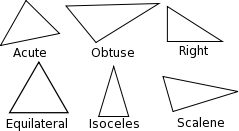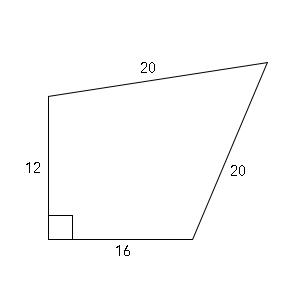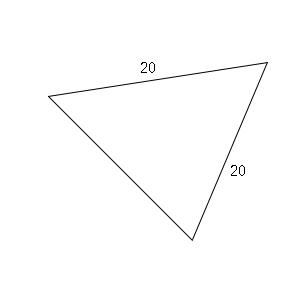# GMAT Quant: Geometry – Triangles

Geometry is all about figures and Triangle is one of the first which one learns in school. Triangles are the most commonly tested topics of GMAT as they present many shortcuts to solve the problem which is vital in this savvy exam. So, let’s know some of the properties of the triangles:

1. Sum of all the internal angles of a triangle is 180°
2. The sum of any two sides of a triangle is always greater than the third side.
3. The difference of any two sides of a triangle is always less than the third side.
4. The longest side of the triangle is always opposite to the largest angle and vice-versa.Here, the largest angle is angle ABC, so the longest side will be opposite to it, which is side AC.

Similarly, the shortest angle is angle CAB, so the shortest side is BC.

1. If two sides of a triangle are equal than its opposite angles are equal.
2. Exterior angle theorem: In a triangle, any exterior angle is equal to sum of the two opposite interior angles.According to this theorem, the sum of angle EFG and angle EGF is equal to angle DEG.

Types of a Triangle:

There are six types of triangle:

1. Acute triangle: Each angle is less than 90°
2. Obtuse triangle: One of the angles is greater than 90°
3. Right triangle: One angle is equal to 90°
4. Equilateral triangle: All the angles are equal to 60° and all sides are equal in length
5. Isosceles triangle: Two angles and two sides of the triangle are equal.
6. Scalene triangle: All the sides and angles are different in measurement.## Triangles: Sample Questions

Let’s solve some questions and understand it properly:

Question:What is the area of the above figure?

Solution:

First of all, let’s cut the figure in two parts since we don’t know the type of quadrilateral it is.

So, after cutting, it becomes,And,Part-1 is a right triangle. So, we can use the Pythagoras theorem to find the third side.

So, it is a Pythagoras Triplet of the form of $(3-4-5) \times 4$, which is 12-16-20

So, the third side is 20.

Hence Area is $\frac{1}{2} \times b \times h$ $\frac{1}{2} \times 12 \times 16 = 96$

Part 2. Now it is an equilateral triangle.

So, its area is $\frac{\sqrt{3}}{4} \times a^{2} = \frac{\sqrt{3}}{4} \times 20^{2} = 100\sqrt{3}$

Total Area = $96 + 100\sqrt{3}$

Read more on GMAT Quant: Special Right Triangles.

We’ll be glad to help you in your GMAT preparation journey. You can ask for any assistance related to GMAT and MBA from us by calling us at +918884544444. You can write to us at gmat@byjus.com.